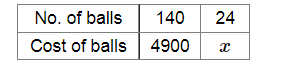# The cost of 140 tennis balls is Rs 4900.

Question:

The cost of 140 tennis balls is Rs 4900. Find the cost of 2 dozen such balls.

Solution:

Let Rs x be the cost of 24 tennis balls.More tennis balls will cost more.

Now, $\frac{140}{4900}=\frac{24}{x}$

$\Rightarrow x=\frac{24 \times 4900}{140}$

$\Rightarrow x=840$

∴ The cost of 2 dozen tennis balls is Rs 840.